# Electronics and Communication Engineering - Signals and Systems

Exercise : Signals and Systems - Section 2
1.
If f=0
XL = 0 and XC = 0
XL = ∞, XC = ∞
XL = 0 and XC = ∞
XL = ∞ and XC = 0
Explanation: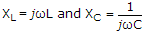.

2.
An ac circuit has an impedance of (2 - j 9) Ω for third harmonic. The impedance for fundamental is
6 - j 27 Ω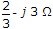2 - j 27 Ω
2 - j 3 Ω
Explanation: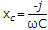.

3.
The ROC of sequence x[n] = (0.8)n ∪[n] + (0.4)n ∪[n]
|z| > 0.8
|z| > 0.4
0.4 < |z| < 0.8
|z| < 0.8
Explanation: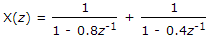ROC ⇒ 1 - 0.8 z-1 < 0 1 - 0.4 z-1

⇒ 1 > 0.8 z-1z > 0.4

or z > 0.8

By combining both condition

ROC because z > 0.4

If you consider z > 0.8 then ROC between the limit 0 to 0.4 excluded.

4.
Pick the odd one
variance
standard deviation
expectation
chebyshev inequality
Explanation:

Because Variance, standard Deviation, Expectation are related to each other.

5.
The sampling of a function f(l) = sin 2pf0t starts from a zero crossing. The signal can be detected if sampling time T is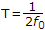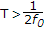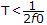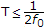Because fs ≤ 2f0, Ts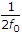.